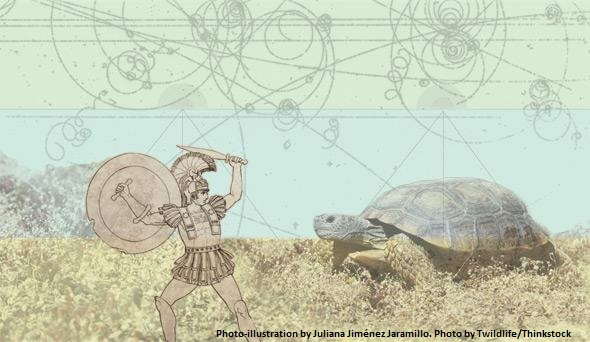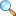# MTH321: Real Analysis I (Spring 2023)At the end of this course the students will be able to understand the basic set theoretic statements and emphasize the proofs’ development of various statements by induction. Define the limit of, a function at a value, a sequence and the Cauchy criterion. Prove various theorems about limits of sequences and functions and emphasize the proofs’ development. Define continuity of a function and uniform continuity of a function, prove various theorems about continuous functions and emphasize the proofs’ development. Define the derivative of a function, prove various theorems about the derivatives of functions and emphasize the proofs’ development. Define a cluster point and an accumulation point, prove the Bolzano-Weierstrass theorem, Rolles’s Theorem, extreme value theorem, and the Mean Value theorem and emphasize the proofs’ development. Define Riemann integral and Riemann sums, prove various theorems about Riemann sums and Riemann integrals and emphasize the proofs’ development.

Questions from Chapter 02

1. A convergent sequence of real number has one and only one limit (i.e. limit of the sequence is unique.)
2. Prove that every convergent sequence is bounded.
3. Suppose that $\left\{ {{s}_{n}} \right\}$ and $\left\{ {{t}_{n}} \right\}$ be two convergent sequences such that $\underset{n\to \infty }{\mathop{\lim }}\,{{s}_{n}}=\underset{n\to \infty }{\mathop{\lim }}\,{{t}_{n}}=s$. If ${{s}_{n}}<{{u}_{n}}<{{t}_{n}}$ for all $n\ge {{n}_{0}}$, then the sequence $\left\{ {{u}_{n}} \right\}$ also converges to $s$.
4. For each irrational number $x$, there exists a sequence $\left\{ {{r}_{n}} \right\}$ of distinct rational numbers such that $\underset{n\to \infty }{\mathop{\lim }}\,\,{{r}_{n}}=x$.

Questions from Chapter 03

1. Prove that if $\sum\nolimits_{n=1}^{\infty }{{{a}_{n}}}$ is convergent then $\underset{n\to \infty }{\mathop{\lim }}\,{{a}_{n}}=0$ but converse is not true.
2. A series $\sum{{{a}_{n}}}$ is convergent if and only if for any real number $\varepsilon >0$, there exists a positive integer ${{n}_{0}}$ such that $\left|\sum_{i=m+1}^{n} a_i \right|<\varepsilon$ $\forall$ $n\ge m>{{n}_{0}}$.
3. Suppose $\sum{{{a}_{n}}}$ and $\sum{{{b}_{n}}}$ are infinite series such that ${{a}_{n}}>0$, ${{b}_{n}}>0$ for all $n$. Also suppose that for a fixed positive number $\lambda$ and positive integer $k$, $a_n<\lambda b_n$ $\forall$ $n\ge k$.
1. If $\sum{{{b}_{n}}}$ is convergent, then $\sum{{{a}_{n}}}$ is convergent.
2. If $\sum{{{a}_{n}}}$ is divergent, then $\sum{{{b}_{n}}}$ is divergent.
4. Prove that every absolute convergent series is convergent, but convers is not true in general.

Questions from Chapter 04

1. Consider the function $f:[0,1]\to \mathbb{R}$ defined as $f(x)=\left\{ \begin{matrix} 0 \text{ if$x$is rational,} \\ 1 \text{ if$x$is irrational.}\end{matrix}\right.$ Show that $\underset{x\to p}{\mathop{\lim }}\,f(x)$, where $p\in [0,1]$ does not exist.
2. If $\underset{x\to c}{\mathop{\lim }}\,f(x)$ exists, then it is unique.
3. A function $f(x)={{x}^{2}}$ is continuous for all $x\in \mathbb{R}$.
4. Suppose $f$ is continuous on $\left[ a,b \right]$ and $f(a)\ne f(b)$, then given a number $\lambda$ that lies between $f(a)$ and $f(b)$, there exist a point $c\in (a,b)$ with $f(c)=\lambda$.

Questions from Chapter 05

1. Prove that a differentiable function is continuous, but the converse is not true.
2. Let $f$ be defined on $\left[ a,b \right]$ and it is differentiable on $(a,b)$. If $f$ has a local maximum at a point $x\in (a,b)$ and if ${f}'(x)$ exist, then ${f}'(x)=0$.
3. Let $f$ be continuous on $[a,b]$and differentiable on $(a,b)$. Then there exists a point $c\in (a,b)$ such that $\frac{f(b)-f(a)}{b-a}={f}'(c)$.
4. If $f$ and $g$ are continuous real valued functions on closed interval $\left[ a,b \right]$ and $f$ and $g$ are differentiable on $\left( a,b \right)$, then there is a point $c\in \left( a,b \right)$ at which $\left[ f(b)-f(a) \right]{g}'(c)=\left[ g(b)-g(a) \right]{f}'(c)$.
• The Real Number System: Ordered Fields. The Field of Reals. The Extended Real Number System. Euclidean Space.
• Numerical Sequences and Series. Limit of a Sequence. Bounded Sequences. Monotone Sequences. Limits Superior and Inferior. Subsequences.
• Limit of a Function and Continuous Functions. Uniform Continuity. Kinds of Discontinuities.
• Derivable and Differentiable Functions. Mean Value theorems. The Continuity of Derivatives. Taylor's theorem.
• Riemann Stieltijes Integrals: Definition, Existence and Properties of the Riemann Integrals. Integral and Differentiation.
Did you know?
• The development of calculus in the 18th century used the entire set of real numbers without having defined them cleanly. The first rigorous definition was given by Georg Cantor in 1871.
• In the 16th century, Simon Stevin created the basis for modern decimal notation, and insisted that there is no difference between rational and irrational numbers in this regard.
• Tuesday, 0830-1000
• Wednesday, 0830-1000

Please download PDF files of the notes given below. To view PDF files, there must be PDF Reader (Viewer) installed on your PC or mobile or smartphone. It can be downloaded from Software section or a user viewing on Android Smartphone may consider EBookDroid to view PDF on their smartphone.

• Chapter 01: Real Number System | Download PDF |View Online
• Chapter 02: Sequences | Download PDF |View Online
• Chapter 03: Series | Download PDF |View Online
• Chapter 04: Limit & Continuity | Download PDF |View Online
• Chapter 05: Differentiation | Download PDF |View Online• Assignment 01 | Download PDF |View Online
• Assignment 02 | Download PDF |View Online
• Assignment 03 | Download PDF |View Online
• Assignment 04 | Download PDF |View OnlinePlease click on View Online to see inside the PDF.

There will be two questions having three parts each. First part of each question will be any definitions, second part will be from questions given below and third part will be related to application of the theory.Questions from Chapter 01

1. Archimedean Property
2. The Density Theorem
3. If $r$ is rational and $x$ is irrational, then $r+x$ is irrational.
4. If $r$ is non-zero rational and $x$ is irrational, then $r\,x$ is irrational.
5. Let $\underline{x}\,,\,\underline{y}\in {{\mathbb{R}}^{k}}$ then $\left| \,\underline{x}\cdot \underline{y}\, \right|\le \left\| \,\underline{x}\, \right\|\,\,\left\| \,\underline{y}\, \right\|$. (Cauchy-Schwarz’s inequality)
6. Suppose $\underline{x}\,,\,\underline{y},\underline{z}\in {{\mathbb{R}}^{k}}$, then prove that
1. $\left\| \,\underline{x}+\underline{y}\, \right\|\le \left\| \,\underline{x}\, \right\|+\left\| \,\underline{y}\, \right\|$.
2. $\left\| \,\underline{x}-\underline{z}\, \right\|\le \left\| \,\underline{x}-\underline{y}\, \right\|+\left\| \,\underline{y}-\underline{z}\, \right\|$.

Questions from Chapter 02

1. A convergent sequence of real number has one and only one limit (i.e. limit of the sequence is unique.)
2. Prove that every convergent sequence is bounded.
3. Suppose that $\left\{ {{s}_{n}} \right\}$ and $\left\{ {{t}_{n}} \right\}$ be two convergent sequences such that $\underset{n\to \infty }{\mathop{\lim }}\,{{s}_{n}}=\underset{n\to \infty }{\mathop{\lim }}\,{{t}_{n}}=s$. If ${{s}_{n}}<{{u}_{n}}<{{t}_{n}}$ for all $n\ge {{n}_{0}}$, then the sequence $\left\{ {{u}_{n}} \right\}$ also converges to $s$.
4. For each irrational number $x$, there exists a sequence $\left\{ {{r}_{n}} \right\}$ of distinct rational numbers such that $\underset{n\to \infty }{\mathop{\lim }}\,\,{{r}_{n}}=x$.
5. A Cauchy sequence of real numbers is bounded.
6. Cauchy’s General Principle for Convergence: A sequence of real number is convergent if and only if it is a Cauchy sequence.

Sample questions related to applications

1. Let $E=\left\{1,\dfrac{1}{2},\dfrac{1}{3},... \right\} \subseteq \mathbb{R}$. Then write the set of upper and lower bounds of $E$.
2. Write subset $E$ of $\mathbb{N}$ such that $\sup E = \inf E$.
3. By defintion, prove that $0$ is even number.
4. Prove that $\left\{1+\dfrac{1}{n} \right\}$ is monotone sequence.
5. Write two subsequence of $\{(-1)^n\}$, which converges to different limits.
6. Write a reason that $\{n+\frac{1}{n} \}$ is not Cauchy sequence.
1. Rudin, W. (1976). Principle of Mathematical Analysis, McGraw Hills Inc.
2. Bartle, R.G., and D.R. Sherbert, (2011): Introduction to Real Analysis, 4th Edition, John Wiley & Sons, Inc.
3. Apostol, Tom M. (1974), Mathematical Analysis, Pearson; 2nd edition.